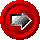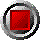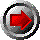CubicSplineInterp(), LinInterp(), and LogLinInterp() functionsCubicSplineInterp(), LinInterp(), and LogLinInterp() functions

CubicSplineInterp(argument list…)

LinInterp(argument list…)

LogLinInterp(argument list…)

These functions return the cubic-spline, linear, or log-linear interpolation of y=f(x) given x, respectively. The cubic-spline interpolation produces a smooth fit through the set of paired points given in xvec and yvec, in the sense that the first and second derivatives of the fitted function are continuous. The functions use the following arguments:

 Argument Description Restrictions x the value of x for which we wish to determine the value of y = f(x) must be within the domain of xvec, that is: min(xvec) <= x <= max(xvec) xvec a vector (or array) of known x values must be in strictly ascending order yvec a vector (or array) of known values of y = f(x) must be same length as xvec

A typical use of this function would be to interpolate an interest rate to an arbitrary date from a given yield curve. In this example, x would be the arbitrary date, xvec an array or yield curve dates, and yvec an array of corresponding interest rates.

© 1995-98 Leap of Faith Research Inc.